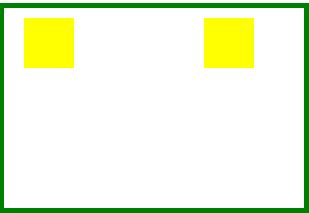# Canvas Hover in HTML5

### Canvas Hover

It is used to add the hover to the elemets to add the touch events

``` <h4>Hover the rectangle to change the color</h4>
<canvas id="mycanvas" width=300 height=200 style="border:5px solid green"></canvas>
<script>
var canvas = document.getElementById("mycanvas"),
context = canvas.getContext("2d"),
rects = [
{
x: 20,
y: 10,
w: 50,
h: 50
},

{
x: 200,
y: 10,
w: 50,
h: 50
}
],
i = 0, r;

while (r = rects[i++])
context.rect(r.x, r.y, r.w, r.h);
context.fillStyle = "blue";
context.fill();

canvas.onmousemove = function (e) {

var rect = this.getBoundingClientRect(),
x = e.clientX - rect.left,
y = e.clientY - rect.top,
i = 0, r;

context.clearRect(0, 0, canvas.width, canvas.height);

while (r = rects[i++]) {

context.beginPath();
context.rect(r.x, r.y, r.w, r.h);
context.fillStyle = context.isPointInPath(x, y) ? "blue":"yellow";
context.fill();
}

};
</script>
```
• In the above code snippet we have defined the` hover` to change the color of the rectangle.  we have canvas element as id as `mycanvas` and width as `300` and height as `200`, border as `5px` solid green
• `var canvas as document.getelement ID` as `mycanvas` and context as defined to the canvas
• We have defined the two rectangles in the with the `x, y`, width and height values
• In the nextline we are defing the` i` value as `o, r,` the `while` condition is used for incrementing the r value with` r++`
• `context.rect(r.x, r.y, r.w, r.h);` - It defines the `(x, y, width, height)` values
• `context.fillStyle = "blue";` - It defines the color of the rectangle color blue
• In the nextline we are having the function   `canvas.onmousemove = function (e) `to define the rectangle  with the `x, y, i, r ` values
• In the nextline we are using the `while` condition for the `increment `of the` i` value with defining the `x,y, width, height` values
• `context.fillStyle = context.isPointInPath(x, y) ? "blue":"yellow"` - The point in path (x,y) value defines the color as blue and when the hover is placed on the rectangle it will change the color as yellow

outputAfter Placing the hover it appears asViews: 30871 | Post Order: 48